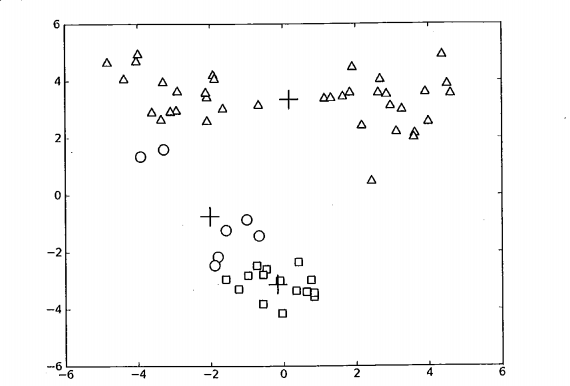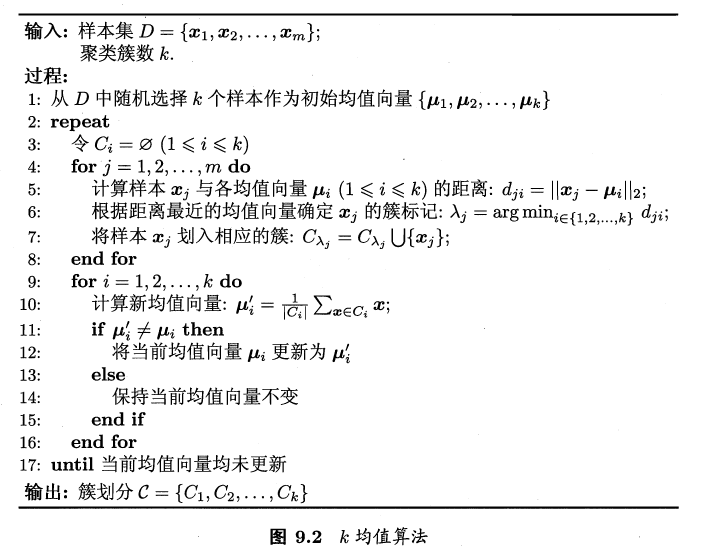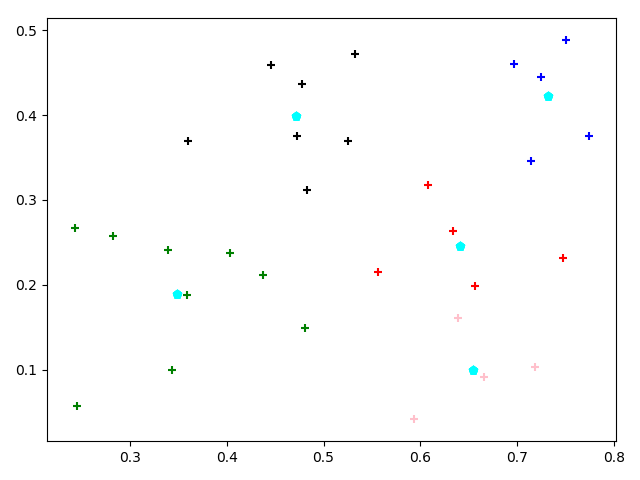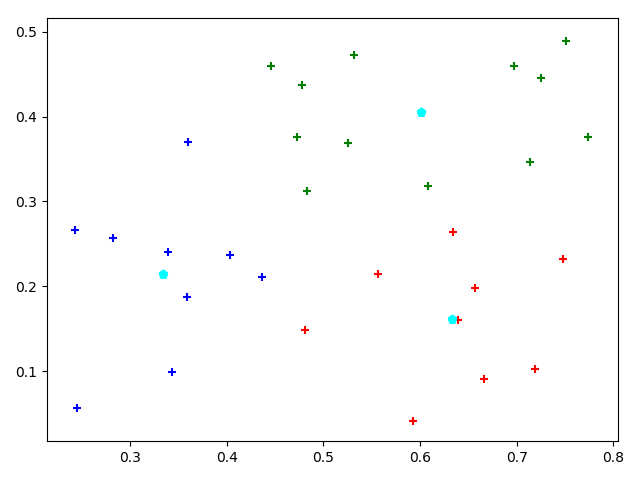2018-07-04 10:38:57 ReXueLaoNanHai 阅读数 335
• ###### 机器学习之概率与统计推断

本课程讲解机器学习算法所需概率和统计推断知识。概率部分包括概率公理及推论、条件概率、贝叶斯公式、随机变量及其概率函数（CDF／pdf）、常用概率分布及其均值、方差；统计推断部分包括大数定律和中心极限定理、极大似然估计、贝叶斯估计，估计的评价、偏差-方差平衡。课程还会讲解假设检验的基本概念。

20165 人正在学习 去看看 AI100讲师

二分k均值算法

K均值聚类算法存在的问题

K均值聚类算法虽然便于理解和实现，但是有其自身的缺点，由于k均值算法初始的k个质心是随机生成的，然后对质心不断的优化，寻找最优的质心以进行聚类，但有时聚类的效果并不是很好，原因就是k均值算法是一种局部最优算法而非全局最优算法，如图对一个包含3个族类的数据进行聚类，由于初始质心的选择问题，当程序更新质心的过程中已经使每条数据的分类都不再变化，程序结束，但是明显数据并没有很好的归类，该质心只是保证每个属于该质心族类的数据到质心距离最小，但本身该质心是不是出现在最好的位置我们并不能保证。为解决上述问题，我们可以引入SSE指标--误差平方和（见系列文章k均值聚类算法中的dataTag），使整个数据集的误差平方和最小这一全局考量可以做到全局的优化。上图中我们把具有最大误差平方的一个类分成两类，为保证总的分类数不变，再把某两个类聚合为一个，具体的合并方法一个是合并距离最近的两个质心，另一个是使合并后的误差平方和最小。

有了这个思路，我们可以逆向思维，因为直接拆分和合并太麻烦，我们可以按照使误差平方和最小的原则，对初始的数据集进行分类（这样没有合并的过程）。

将所有点看成一个类别

当类别数小于k

对每一个类

计算总的误差平方和

在当前类内进行k均值聚类，k的值为2

计算将该类一分为二后总的误差平方和

选择使得总的误差平方和最小的划分类进行划分

def biKmeans(dataSet, k, distMeas=disEclud):

m = shape(dataSet)

clusterAssment = mat(zeros((m,2)))

centroid0 = mean(dataSet, axis=0).tolist()

centList =[centroid0] #create a list with one centroid

for j in range(m):#calc initial Error

clusterAssment[j,1] = distMeas(mat(centroid0), dataSet[j,:])**2

while (len(centList) < k):

lowestSSE = inf

for i in range(len(centList)):

ptsInCurrCluster = dataSet[nonzero(clusterAssment[:,0].A==i),:]#get the data points currently in cluster i

centroidMat, splitClustAss = kMeans(ptsInCurrCluster, 2, distMeas)

sseSplit = sum(splitClustAss[:,1])#compare the SSE to the currrent minimum

sseNotSplit = sum(clusterAssment[nonzero(clusterAssment[:,0].A!=i),1])

print( "sseSplit, and notSplit: "+sseSplit+","+sseNotSplit)

if (sseSplit + sseNotSplit) < lowestSSE:

bestCentToSplit = i

bestNewCents = centroidMat

bestClustAss = splitClustAss.copy()

lowestSSE = sseSplit + sseNotSplit

bestClustAss[nonzero(bestClustAss[:,0].A == 1),0] = len(centList) #change 1 to 3,4, or whatever

bestClustAss[nonzero(bestClustAss[:,0].A == 0),0] = bestCentToSplit

print('the bestCentToSplit is: '+bestCentToSplit)

print('the len of bestClustAss is: '+ len(bestClustAss))

centList[bestCentToSplit] = bestNewCents[0,:].tolist()#replace a centroid with two best centroids

centList.append(bestNewCents[1,:].tolist())

clusterAssment[nonzero(clusterAssment[:,0].A == bestCentToSplit),:]= bestClustAss#reassign new clusters, and SSE

return mat(centList), clusterAssment

首先shape（）函数得到总数据集的行数；

为记录数据集每条数据所属分类和距离质心的误差平方和生成一个m行2列的矩阵；

先用mean（）函数得到整个数据集的质心（每个特征的平均值），centList记录质心；

计算数据到质心的距离；初始误差平方和定义为无穷大；从数据集中选出某一类（i类）的数据保存到ptsInCurrCluster ，对该类数据进行2分k均值算法将数据一分为二；

计算新划分出的两类数据的误差平方和；计算数据集中不属于i类的数据误差平方和，因为当前只是选出i类数据进行一分为二，对划分后的数据并没有改变其在原数据集中的分类或误差值，所以不等于i的就是剩余的所有数据（这一点我自己看的时候总是以为已经划分了，认为筛选不出剩余的数据。。。），计算总和，如果误差比原来小了就记录下本次分类是在哪个类（i）上进行的；

记录新的质心以及新的分类和误差等；for循环结束，遍历完所有的族类后，几个best..变量中存的就是使误差平方和最小的分类记录；然后开始进行划分（此处才真正执行划分！）；

在执行2分k均值的i类上：a对于新划分出的1类数据给其分配的标签是族类的长度值，即扩展的类名，对于新划分出的0类数据仍然保持其原来分类标签，即还是i；b语句centList[bestCentToSplit] = bestNewCents[0,:].tolist()将原来被划分的i类处质心更新为新划分出的0类质心，语句centList.append(bestNewCents[1,:].tolist())，将新划分出的1类质心增加到质心列表里，b部分更新了质心；c最后选出执行划分的数据分类及误差平方记录表，将其更新为新的类名和误差，a部分进行了命名。

k均值算法 机器学习 相关内容

2019-11-29 15:06:30 weixin_41848012 阅读数 28
• ###### 机器学习之概率与统计推断

本课程讲解机器学习算法所需概率和统计推断知识。概率部分包括概率公理及推论、条件概率、贝叶斯公式、随机变量及其概率函数（CDF／pdf）、常用概率分布及其均值、方差；统计推断部分包括大数定律和中心极限定理、极大似然估计、贝叶斯估计，估计的评价、偏差-方差平衡。课程还会讲解假设检验的基本概念。

20165 人正在学习 去看看 AI100讲师

#### 【机器学习】西瓜书_周志华，习题9.4，编程实现k均值算法

##### 1. 核心算法：k均值算法作为原型聚类算法的一种，其主要目标最小化平方误差，通过不断迭代更新均值向量，从而得到新的簇分类。（使簇内距离最小）

##### 2. 具体实现：
``````# -*- coding: utf-8 -*-
#author: w61

import random
import math
import matplotlib.pyplot as plt

class Kmeans():
def __init__(self,k,mode):
self.datasets = []
self.vector = 0                             #记录数据集的维数
self.k = k                                      #聚类簇数
self.C = [[] for i in range(k)]                  #簇
self.mode = 0                               #初始化方式
self.mean_vector = []                             #均值向量
self.update_flag = 0                        #记录均值向量是否改变
self.count = 0                              #记录循环次数
self.max_count = 500                        #最大运行轮数

"""
读取数据集
:param file_name:数据集的路径
:return:null
"""
with open(file_name) as f:
for contents in f:
contents = contents.replace('\n','')
content = contents.split(' ')
self.datasets.append(content[1:])
self.vector = len(self.datasets)

def Inialize(self):
"""
初始化均值向量
:return:null
"""
if self.mode == 0:
means = random.sample(range(0,len(self.datasets)),self.k)
for mean in means:
self.mean_vector.append(self.datasets[mean])

def GetDistance(self,x,y):
"""
计算两个向量(x和y)之间的距离
:param x:
:param y:
:return: dis 距离
"""
dis = 0
for i in range(self.vector):
dis += pow((float(x[i])-float(y[i])),2)
dis = math.sqrt(dis)
return dis

def Clustering(self):
"""
划分簇
:return:null
"""
self.C = [[] for m in range(self.k)]   #每一次都要先把C置零
for j in range(0,len(self.datasets)):
min_dis = 0
min_index = 0
for i in range(self.k):
dis = self.GetDistance(self.datasets[j],self.mean_vector[i])
if min_dis == 0 or dis < min_dis:
min_dis = dis
min_index = i
self.C[min_index].append(j)

def Update(self):
"""
更新每个簇的均值向量
:return:
"""
self.update_flag = 0
for i in range(self.k):
means= []
for h in range(self.vector):
sum = 0
for j in self.C[i]:
sum += float(self.datasets[j][h])
if len(self.C[i]) == 0:
mean = 0
else:
mean = sum / len(self.C[i])
means.append(mean)
if means == self.mean_vector[i]:
continue
else:
self.mean_vector[i] = list(means)
self.update_flag = 1

def Plot(self):
"""
绘图
:return:
"""
fig = plt.figure()
colors = ['red','blue','green','black','pink','brown','gray']

for i in range(self.k):
for j in self.C[i]:
x = float(self.datasets[j])
y = float(self.datasets[j])
plt.scatter(x,y,color = colors[i],marker='+')

for i in range(self.k):
for j in self.mean_vector:
x = float(j)
y = float(j)
plt.scatter(x,y,color = 'cyan',marker='p')
plt.show()

def Process(self):
"""
执行函数
:return:
"""
self.Inialize()
while self.count <= self.max_count:
self.Clustering()
self.Update()
if self.update_flag == 0:
break
self.count += 1
print('k-means分类结果如下：')
print(self.C)
self.Plot()

if __name__ == "__main__":
kmeans = Kmeans(5,0)
kmeans.Process()
``````

（1）5分类：（2）3分类：## 附:

data.txt数据集：
1 0.697 0.460
2 0.774 0.376
3 0.634 0.264
4 0.608 0.318
5 0.556 0.215
6 0.403 0.237
7 0.481 0.149
8 0.437 0.211
9 0.666 0.091
10 0.243 0.267
11 0.245 0.057
12 0.343 0.099
13 0.639 0.161
14 0.657 0.198
15 0.360 0.370
16 0.593 0.042
17 0.719 0.103
18 0.359 0.188
19 0.339 0.241
20 0.282 0.257
21 0.748 0.232
22 0.714 0.346
23 0.483 0.312
24 0.478 0.437
25 0.525 0.369
26 0.751 0.489
27 0.532 0.472
28 0.473 0.376
29 0.725 0.445
30 0.446 0.459

k均值算法 机器学习 相关内容

2019-11-02 15:30:04 Mr_Sherlock 阅读数 26
• ###### 机器学习之概率与统计推断

本课程讲解机器学习算法所需概率和统计推断知识。概率部分包括概率公理及推论、条件概率、贝叶斯公式、随机变量及其概率函数（CDF／pdf）、常用概率分布及其均值、方差；统计推断部分包括大数定律和中心极限定理、极大似然估计、贝叶斯估计，估计的评价、偏差-方差平衡。课程还会讲解假设检验的基本概念。

20165 人正在学习 去看看 AI100讲师

# k均值算法

二、遍历数据集中的每个样本，求出数据集中每个样本关于第一步随机选出的k个初始向量的欧式距离，然后在这些距离中选出最小的距离，并将这个样本进行标记，标记的内容是这个样本属于哪一簇。

三、这一步基于第二步已经得出了数据集D中的每个样本属于哪个簇。将每个簇中的样本进行求均值运算，这样就会得到k个新的初始向量。

四、进行迭代运算，反复重复第一步到第三步的操作，直到迭代得到的新的初始向量和上一次迭代得到的初始向量相同，算法结束。

``````0.697 0.460
0.774 0.376
0.634 0.264
0.608 0.318
0.556 0.215
0.403 0.237
0.481 0.149
0.437 0.211
0.666 0.091
0.243 0.267
0.245 0.057
0.343 0.099
0.639 0.161
0.657 0.198
0.360 0.370
0.593 0.042
0.719 0.103
0.359 0.188
0.339 0.241
0.282 0.257
0.748 0.232
0.714 0.346
0.483 0.312
0.478 0.437
0.525 0.369
0.751 0.489
0.532 0.472
0.473 0.376
0.725 0.445
0.446 0.459``````
``````//原始聚类的k均值算法
#include<iostream>
#include<cmath>

using namespace std;

struct Dataset{
float md;//密度
float sugar;//含糖量
int flag;//作为标记，显示属于哪个簇
};

//计算欧式距离
//6->(0.403;0.237) 12->(0.343;0.099) 27->(0.532;0.472)
float euclidean(float ax,float ay,float bx,float by)
{
float a=pow((bx-ax),2);
float b=pow((by-ay),2);
float result =sqrt(a+b);
return result;
}
//找出最小值
int findMin(float a,float b,float c)
{
int flag=1;
float min=a;
if(b<min)
{
min=b;
flag=2;
}
if(c<min)
{
min=c;
flag=3;
}
return flag;
}

int main()
{
int m,k,i,n1=0,n2=0,n3=0;//m为数据集的个数、k为划分的簇的个数 ,n1,n2,n3分别为每个簇内样本的数量
float x1=0.403,y1=0.237,x2=0.343,y2=0.099,x3=0.532,y3=0.472; //初始的三个均值向量的值
float dist1,dist2,dist3;
cin>>m;
Dataset ds[m+1];
for(i=1;i<=m;++i)
{
cin>>ds[i].md>>ds[i].sugar;
}
//对样本进行初次划分簇,给每个样本加上标记
for(i=1;i<=m;++i)
{
dist1 = euclidean(ds[i].md,ds[i].sugar,x1,y1);
dist2 = euclidean(ds[i].md,ds[i].sugar,x2,y2);
dist3 = euclidean(ds[i].md,ds[i].sugar,x3,y3);
int min = findMin(dist1,dist2,dist3);//找到欧式距离最小的一个下标，然后将该样本的标记设为该簇的下标
if(min==1)
{
n1++;
}
if(min==2)
{
n2++;
}
if(min==3)
{
n3++;
}
ds[i].flag = min;
}
//求出三个新的均值向量
float s1x=0,s1y=0,s2x=0,s2y=0,s3x=0,s3y=0;
for(i=1;i<=m;++i)
{
if(ds[i].flag==1)
{
s1x+=ds[i].md;
s1y+=ds[i].sugar;
}
if(ds[i].flag==2)
{
s2x+=ds[i].md;
s2y+=ds[i].sugar;
}
if(ds[i].flag==3)
{
s3x+=ds[i].md;
s3y+=ds[i].sugar;
}
}
float nx1,ny1,nx2,ny2,nx3,ny3;
//新的均值向量的坐标
nx1=s1x/n1;
nx2=s2x/n2;
nx3=s3x/n3;
ny1=s1y/n1;
ny2=s2y/n2;
ny3=s3y/n3;
cout<<"m1("<<nx1<<","<<ny1<<") m2("<<nx2<<","<<ny2<<") m3("<<nx3<<","<<ny3<<")"<<endl;
return 0;
}``````

k均值算法 机器学习 相关内容

2017-06-04 18:03:23 program_developer 阅读数 1763
• ###### 机器学习之概率与统计推断

本课程讲解机器学习算法所需概率和统计推断知识。概率部分包括概率公理及推论、条件概率、贝叶斯公式、随机变量及其概率函数（CDF／pdf）、常用概率分布及其均值、方差；统计推断部分包括大数定律和中心极限定理、极大似然估计、贝叶斯估计，估计的评价、偏差-方差平衡。课程还会讲解假设检验的基本概念。

20165 人正在学习 去看看 AI100讲师

9.4 试编程实现k均值算法，设置三组不同的k值、三组不同初始中心点，在西瓜数据集4.0上进行试验比较，并讨论什么样的初始中心有利于取得好结果。

K-means算法：初始随机的中心点不同会导致算法的迭代次数与最终结果有很大的不同。一般来说，初始的中心点越集中且越靠近边缘，则会使得迭代次数更多。初始中心点越分散，迭代次数越少，结果越好。

K=3的结果：```x = xlsread('E:\machine_learning\Machine-Learning-ZhouZhiHua\9.4\watermelon4.0.xlsx', 'Sheet1', 'A1:B30');
[m,n]=size(x);    %m表示行、n表示列
k=3;%设置k值,即要聚类的个数
u=x(randperm(m,k),:);  %随机均值
while 1
c=zeros(k,30);    %将各类集合清空
nums=zeros(k,1);
%对所有样本遍历，选择最近的集合
for i=1:m
mind=100000;   %存放最短的距离
minl=0;        %x的族标记
for j=1:k
d=norm(x(i,:)-u(j,:));   %计算样本x到各均值向量的距离
if(d<mind)
mind=d;
minl=j;
end
end
nums(minl)=nums(minl)+1;   %相应族的聚类个数
c(minl,nums(minl))=i;      %
end
%计算两次均值差异，并更新均值
up=zeros(k,2);
for i=1:k
for j=1:nums(i)
up(i,:)=up(i,:)+x(c(i,j),:);
end
up(i,:)=up(i,:)/nums(i);
end
%迭代结束的条件
delta_u=norm(up-u);
if(delta_u<0.001)
break;
else
u=up;
end
end

%各类使用不同的符号绘制
ch='o*+.>';

for i=1:k
%绘制类中的点
plot(x(c(i,1:nums(i)),1),x(c(i,1:nums(i)),2),ch(i));
hold on;
tc=x(c(i,1:nums(i)),:);       %同族样本所有数据
%计算类凸包并画线
%chl=convhull(tc(:,1),tc(:,2));   %Octave中convhull(x,y)必须有两个参数
%在Matlab中使用
chl=convhull(tc);
line(tc(chl,1),tc(chl,2))
hold on;
end

xlabel('密度');
ylabel('含糖率');
title('K-means'); ```

k=2k=4k均值算法 机器学习 相关内容

2017-06-28 21:53:22 Lunar112 阅读数 412
• ###### 机器学习之概率与统计推断

本课程讲解机器学习算法所需概率和统计推断知识。概率部分包括概率公理及推论、条件概率、贝叶斯公式、随机变量及其概率函数（CDF／pdf）、常用概率分布及其均值、方差；统计推断部分包括大数定律和中心极限定理、极大似然估计、贝叶斯估计，估计的评价、偏差-方差平衡。课程还会讲解假设检验的基本概念。

20165 人正在学习 去看看 AI100讲师

# 一. K均值算法介绍及实现

K均值算法（K-means)是无监督学习中的一种，其算法简洁容易实现，且Sklearn包中也提供了相应的模块可以直接调用。在<<机器学习实战>>书中，也对该算法进行了介绍。

k 均值 是 发现 给定 数据 集 的 k 个 簇 的 算法。 簇 个数 k 是 用户 给定 的， 每一个 簇 通过 其 质 心（ centroid）， 即 簇 中 所 有点 的 中心 来 描述。*: k为最终簇（也即质心）的数量

– 对数 据 集中 的 每个 数据 点
— 对 每个 质 心
— 计算 质 心 与 数据 点 之间 的 距离
— 将 数据 点 分配 到 距 其 最 近的 簇
– 对 每一个 簇， 计算 簇 中 所 有点 的 均值 并将 均值 作为 质 心

``````from numpy import *
import matplotlib.pyplot as plt
from sklearn.cluster import KMeans

'''
@fun: 载入文件，并将文件里的数据转化为float型
@input: fileName --- 文件地址
@return: list型的数据
注意：这里的文件是原始数据集。每一行对应了一个点。每一行有两个数据，第一个为x坐标，第二个为y坐标。两个数据由Tab分开
'''
dataMat = []
fr = open(fileName)
curLine = line.strip().split('\t') #按tab来分隔数据
fltLine = list(map(float,curLine))
dataMat.append(fltLine)
return dataMat

def distEclud(vecA, vecB):
#计算两个向量的欧式公式。将每个向量看成一个点。
return sqrt(sum(power(vecA - vecB, 2)))

def randCent(dataSet, k):
'''
@fun: 产生K个随机的质心
@input: dataSet --- 数据集
k --- 随机质心的数量
@return: matrix. 每一行为一个随机质心的坐标向量
'''
n = shape(dataSet)
centroids = mat(zeros((k,n)))
for j in range(n):
minJ = min(dataSet[:,j])
maxJ=max((dataSet[:,j]))
rangeJ= float(maxJ- minJ)
centroids[:,j] = mat(minJ + rangeJ * random.rand(k,1))
return centroids

def kMeans(dataSet, k, distMeas=distEclud, createCent=randCent):
'''
@fun: k-mean算法实现
@input: dataSet --- 原始数据集
k --- 质心数量
disMeas --- 距离计算的方法，默认为distEclud
createCent --- 生成随机质心的方法，默认为randCent
@return
'''
m = shape(dataSet)
clusterAssment = mat(zeros((m,2)))

centroids = createCent(dataSet, k)
clusterChanged = True
while clusterChanged:
clusterChanged = False
for i in range(m):
minDist = inf; minIndex = -1
for j in range(k):
distJI = distMeas(centroids[j,:],dataSet[i,:])
if distJI < minDist:
minDist = distJI; minIndex = j
if clusterAssment[i,0] != minIndex: clusterChanged = True
clusterAssment[i,:] = minIndex,minDist**2 #注意clusterAssment每行有两个元素，一个是质心序号，另一个是到质心的距离
# print (centroids)
for cent in range(k):
ptsInClust = dataSet[nonzero(clusterAssment[:,0].A==cent)]
centroids[cent,:] = mean(ptsInClust, axis=0)
return centroids, clusterAssment

def plotKMeanFig(dataMat,clusterAssment,centroids,ax):
'''
@fun: 绘制图形
@para: dataMat --- 原始数据点
clusterAssment --- 分类的信息。第0列是数据簇的序号
centroids --- 质心的列表。
ax --- figure里的子图
'''

#绘制数据点
m=len(centroids)
for i in range(m):
xi=dataMat[nonzero(clusterAssment[:,0]==i),0].A1
yi=dataMat[nonzero(clusterAssment[:,0]==i),1].A1
ax.scatter(xi,yi,s=10)

#绘制质心
xCentroids=[co for co in centroids]
yCentroids=[co for co in centroids]
ax.scatter(xCentroids,yCentroids,color='green',s=30,marker='^')

#调用plotKMeansFig()函数就可以绘制出图形
plotKMeansFig()``````

# 二. 改进版本的K均值算法–二分K均值算法

*k为最终簇（也即质心）的数量

– 对于 每一个 簇
— 计算 总 误差
— 在给 定的 簇 上面 进行 K 均值 聚 类（ K= 2）
— 计算 将 该 簇 一分为二 之 后的 总 误差
– 选择 使得 误差 最小 的 那个 簇 进行 划分 操作，划分实施后，簇的数目+1

``````def biKmeans(dataSet, k, distMeas=distEclud):
'''
@fun: k-mean算法实现
@input: dataSet --- 原始数据集
k --- 质心数量
disMeas --- 距离计算的方法，默认为distEclud
@return mat(centList) --- 质心的坐标矩阵
clusterAssment --- 每个点的分类结果矩阵。第0列为质心（簇）的序号，第1列为与质心的距离的平方
'''
#增加以下代码，以便绘制图形
fig=plt.figure()
axList=[ax1,ax2,ax3,ax4]

m = shape(dataSet)
clusterAssment = mat(zeros((m,2)))
centroid0 = mean(dataSet, axis=0).tolist() #按行求平均值
centList =[centroid0] #创建质心的列表，并用一开始得到的平均值初始化
for j in range(m):#计算初始的误差
clusterAssment[j,1] = distMeas(mat(centroid0), dataSet[j,:])**2
plotKMeanFig(dataSet,clusterAssment,centList,axList)
figNo=1 #子图的序号
while (len(centList) < k):
lowestSSE = inf
for i in range(len(centList)):
#get the data points currently in cluster i。注意这里nonzero的用法，nonzero函数会返回一个包含两个元素的list。第0个代表了非零元素所在的行号
ptsInCurrCluster = dataSet[nonzero(clusterAssment[:,0].A==i),:]
centroidMat, splitClustAss = kMeans(ptsInCurrCluster, 2, distMeas)
sseSplit = sum(splitClustAss[:,1])#compare the SSE to the currrent minimum
sseNotSplit = sum(clusterAssment[nonzero(clusterAssment[:,0].A!=i),1])
# print ("sseSplit, and notSplit: ",sseSplit,sseNotSplit)
if (sseSplit + sseNotSplit) < lowestSSE:
bestCentToSplit = i
bestNewCents = centroidMat
bestClustAss = splitClustAss.copy()
lowestSSE = sseSplit + sseNotSplit
bestClustAss[nonzero(bestClustAss[:,0].A == 1),0] = len(centList)
bestClustAss[nonzero(bestClustAss[:,0].A == 0),0] = bestCentToSplit
# print ('the bestCentToSplit is: ',bestCentToSplit)
# print ('the len of bestClustAss is: ', len(bestClustAss))
centList[bestCentToSplit] = bestNewCents[0,:].tolist()#将进一步划分的质心，替换为划分完成的两个新质心
centList.append(bestNewCents[1,:].tolist())
clusterAssment[nonzero(clusterAssment[:,0].A == bestCentToSplit),:]= bestClustAss#reassign new clusters, and SSE
plotKMeanFig(dataSet,clusterAssment,centList,axList[figNo])
figNo+=1
plt.show()
return mat(centList), clusterAssment

def plotKMeanFig(dataMat,clusterAssment,centroids,ax):
'''
@fun: 绘制图形
@para: dataMat --- 原始数据点
clusterAssment --- 分类的信息。第0列是数据簇的序号
centroids --- 质心的列表。
ax --- figure里的子图
@return： N/A
'''
#绘制数据点
m=len(centroids)
for i in range(m):
xi=dataMat[nonzero(clusterAssment[:,0]==i),0].A1
yi=dataMat[nonzero(clusterAssment[:,0]==i),1].A1
ax.scatter(xi,yi,s=10)

#绘制质心
xCentroids=[co for co in centroids]
yCentroids=[co for co in centroids]
ax.scatter(xCentroids,yCentroids,color='green',s=30,marker='^')

#调用biKmeans()函数就可以绘制出图形
dataMat=....   #写入你自己的数据
k=4   #质心的数量
biKmeans(dataMat，k)``````# 三. SKlearn库中的K-Means

Sklearn库中也给出了K-Means算法的相关模块。Sklearn库采用的办法是采用不同的K质心初始值，来进行多次的K-Means算法计算，最后选用结果最好的初始值结果。通过这样的方法可以避免得到一个局部最优而非全局最优的结果。

sklearn.cluster.Kmeans()类由以下几个重要的参数：
n_clusters： 质心的数量，默认为8
max_iter： 单个Kmeans算法最大迭代次数，默认为300
n_init： 随机初始质心的次数。每次产生随机初始质心后都会进行一次Kmeans运算。最终得到的结果，是这里面结果最好的随机初始质心的运算结果。默认为10。将该参数增大可以减少得到局部最优而非全局最优的概率。不过会延长运行时间。

``````
def KMeanswithSKlearn():
myKMeans=KMeans(n_clusters=4,init='k-means++')
myKMeans.fit(dataMat.A)
labels=myKMeans.labels_
labelMat=mat(labels).T
clusterList=myKMeans.cluster_centers_
fig=plt.figure()
plotKMeanFig(dataMat,labelMat,clusterList,ax)
plt.show()

def plotKMeansFig():
centroids, clusterAssment=kMeans(dataMat, 4, distMeas=distEclud, createCent=randCent)
centroidsList=centroids.A
fig=plt.figure()
plotKMeanFig(dataMat,clusterAssment,centroidsList,ax)
plt.show()

#直接调用plotKMeansFig()就可以绘制出图形
plotKMeansFig()
``````k均值算法 机器学习 相关内容